# Calculate the number of moles of iron in a sample containing 10 22 atoms of iron

1. Calculate the number of moles of iron in a sample containing 10 22 atoms of iron.
2. Calculate the number of moles in 71g of chlorine. [Atomic mass ofCl= 35.5 u]
1. Number of moles of iron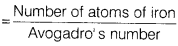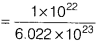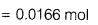2. Molecular mass of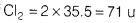Number of moles of Cl =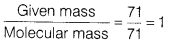∴ 71 g of Cl2 = 1 moj